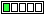All about flooble | fun stuff | Get a free chatterbox | Free JavaScript | Avatarsperplexus dot infoDon't be confused (Posted on 2016-11-14)If x satisfy the equation x^4 - x^2 + 1 = 0 , find the value of x^5 + 1/x

 No Solution Yet Submitted by Danish Ahmed Khan No RatingComments: ( Back to comment list | You must be logged in to post comments.)going complex Comment 4 of 4 |x^2=sqrt(3)/2 + i/2=cos 60+jsin 60 (one of 2 options)

x^4= cos 120+jsin 120                    (numbers of degrees)

x=cos 30 +jsin 30 (one of 2 options)

x^5= x^4*x= cos 150 + jsin 150 =A

1/x= cos 30 - jsin 30=B

A+B= -COS 30 +COS 30 +JSIN 30-JSIN 30=0

QED

I did not bother to show all 4 possibilities...

 Posted by Ady TZIDON on 2016-11-15 14:55:14Please log in:

 Search: Search body:
Forums (1)